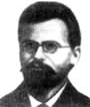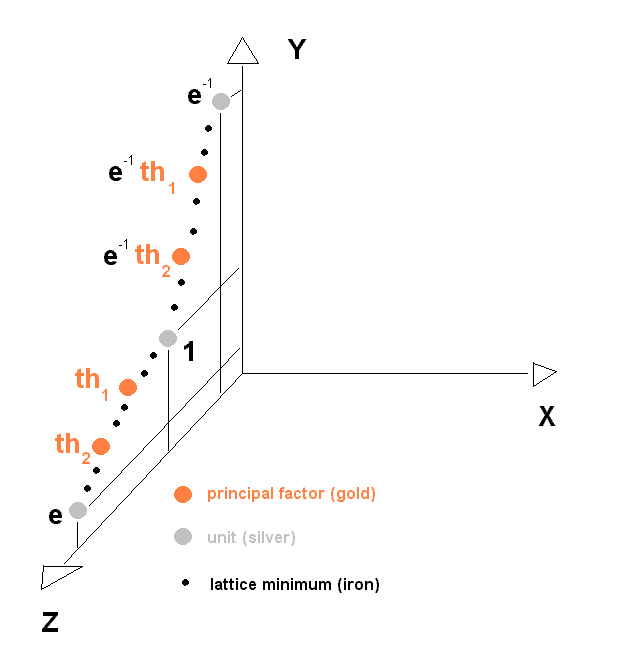# of Karim Belabas, Aïssa Derhem, and Daniel C. Mayer

 * On these pages, we present most recent results of our joint research, directly from the lab. * Basic bibliography: K. Belabas, A fast algorithm to compute cubic fields, Math. Comp. 66 (1997), 1213-1237 A. Derhem, Capitulation dans les extensions quadratiques de corps de nombres cubiques cycliques, Thèse de doctorat, Université Laval, Québec, 1988 D. C. Mayer, Multiplicities of dihedral discriminants, Math. Comp. 58 (1992), 831-847 and S55-S58 * E-mail addresses: Karim.Belabas@math.u-psud.fr aderhem@yahoo.fr danielmayer@algebra.at *

 Exotic pure cubic fields (2002/03/20) Dan (02/03/20): The statistical results presented previously have shown that Principal Factorization Type Beta is clearly the dominating type of pure cubic fields. Therefore, in 77% of the fields L = Q(R^1/3) with R = m*n^2 we can find a non-unital and non-radical primitive generator a of an ambiguous principal ideal (a) = aO_L, which is invariant under the relative automorphisms of the normal field N of L over its quadratic subfield K = Q((-3)^1/2), i. e., a primitive algebraic integer of L, whose norm N(a) divides the square r^2 of the product of all totally ramified rational primes, (*) r = 3*m*n, if R congruent 2,4,5,7 (mod 9) (DT 1B), (**) r = m*n, if 3 | R (DT 1A) or R congruent 1,8 (mod 9) (DT 2), but N(a) differs from 1, m*n^2, and m^2*n. We can even find a generator a of such a so called Differential Principal Factor (principal ideal divisor (a) of the relative different of N | K) between the bounds 1 < a < e, where e denotes the fundamental unit of L. Then we get a representation a^3 / N(a) = e or e^2, which can simplify the process of determining the fundamental unit e ,,. The 2 minimal norms in the orbit of 6 principal factors  are relatively small, in general. In most of the cases, they do not exceed the Minkowski bound (approximately 3.3*m*n). Hence there is a considerable probability to find them along the VORONOI Highway Min(O), i. e., the chain 1 < n(1) < n^2(1) < ... of successive neighboring (adjacent) lattice minima in the discrete geometric image of an order O (e. g., the maximal order O_L) of L.The group U of units of L acts on Min(O) and causes a disjoint decomposition of Min(O) into finitely many U-orbits Min(O) = union_{i = 0}^{p-1} n^i(1)U one of the U-orbits (for i = 0) being the unit group U itself and p denoting the period length with the property n^p(1) = e, the fundamental unit. Indeed, it has been proved for extensive classes of pure cubic fields, that M = #{ 0 < i < p | N(n^i(1)) divides r^2 } = 2, i. e., there exist two chain positions 0 < j < k < p on the VORONOI Highway, empirically approximately at j = p*1/3 and k = p*2/3 in the first primitive period, occupied by principal factors theta1 = n^j(1) and theta2 = n^k(1) with theta1^3 / N(theta1) = e and theta2^3 / N(theta2) = e^2. Hugh C. Williams has shown this fact for the maximal order O = O_L of DT 1A fields with radicands R congruent 0,3,6 (mod 9) in , and I myself observed that his proof holds also for the maximal order O = O_L of DT 1B fields with R congruent 2,4,5,7 (mod 9) and non-escalatory orbit of principal factors (norms coprime to 3) and for the suborder O = Z + Z(m*n^2)^1/3 + Z(m^2*n)^1/3 of DT 2 fields with R congruent 1,8 (mod 9) .The rare PFT Beta fields of DT 2 and of DT 1B with escalatory orbit of principal factors (norms divisible by 3), where the primitive period of O_L contains only one, resp. even none of the principal factors are called half-exotic (M = 1) fields, resp. exotic (M = 0) fields ,. The following tables give the detailed statistics of PFTs and of exotic fields. Dan (02/03/19): Population of the Principal Factorization Types in fields of DT 1A: PFT Alpha (relatively ambiguous principal ideals): 35759 fields (14.06 %) PFT Beta (absolutely ambiguous principal ideals): 209589 fields (82.43 %) PFT Gamma (some unit in the normal field has norm [-1+(-3)^1/2]/2): 8906 fields (3.50 %) All fields of PFT Beta are non-exotic with M = 2. Population of the PFTs in fields of DT 1B: PFT Alpha: 61272 fields (16.03 %) PFT Beta: 320959 fields (83.97 %) PFT Gamma: impossible PFT for DT 1B Population of Orbit Types among fields of DT 1B and PFT Beta: Escalatory Orbit: 233776 fields (72.84 %) Non-Escalatory Orbit: 87183 fields (27.16 %) (Here, we have a conceptual contradiction, since in fact escalatory orbits are more frequent.) All fields of PFT Beta with non-escalatory orbit are non-exotic with M = 2. Population of Exotic Fields among fields of DT 1B and PFT Beta with Escalatory Orbit: M = 0: 582 exotic fields (0.25 %) M = 1: 22740 half-exotic fields (9.73 %) M = 2: 210454 non-exotic fields (90.02 %) Population of the PFTs in fields of DT 2: PFT Alpha: 66499 fields (34.80 %) PFT Beta: 104886 fields (54.88 %) PFT Gamma: 19730 fields (10.32 %) Population of Exotic Fields among fields of DT 2 and PFT Beta: M = 0: 140 exotic fields (0.13 %) M = 1: 338 half-exotic fields (0.32 %) M = 2: 104408 non-exotic fields (99.54 %) Dan (02/03/20): Bibliography: